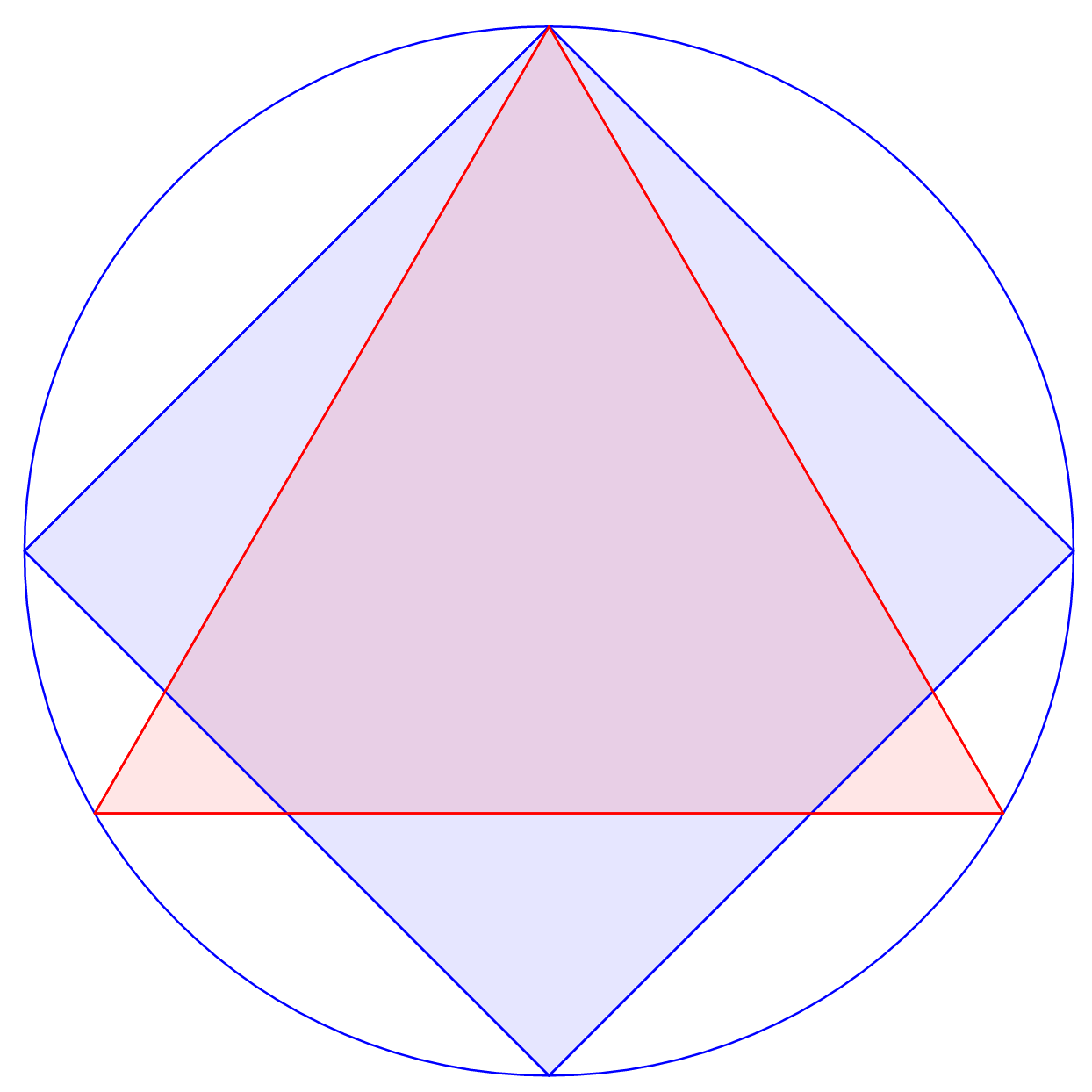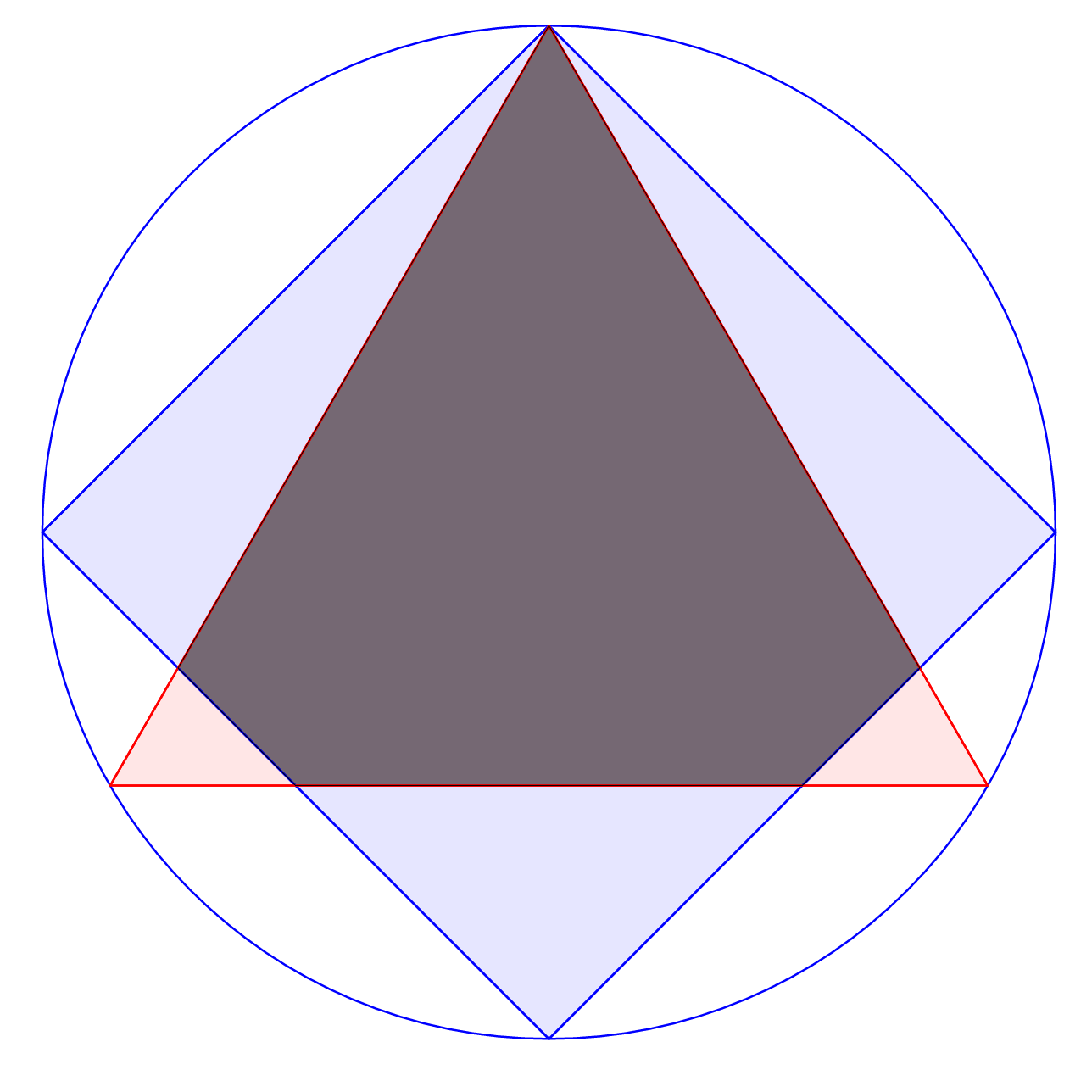# SCAA Logo

Geometry Level 4The figure shows a unit circle with a square and an equilateral triangle inscribed in it.

The square and equilateral triangle share a vertex.The overlapping area of the square and triangle can be expressed as $a\sqrt{b}+\dfrac{c}{d},$ where $a,b,c$ and $d$ are integers with $b$ square-free and $c,d$ coprime.

Find $ab+cd$.

×